# How to make a line follower robot using Arduino and L298N | 4 – way line tracking sensor module

Hello, welcome back. In this tutorial, we will learn how to make a line follower robot using Arduino. Also, this project mainly uses the 4-way line tracking sensor module. In a previous article, we presented how to make a line follower robot. For that, click on this link. Also, this tutorial is presented step by step so that you can create your own line follower robot by the end of this tutorial.

### What is the 4-way line tracking sensor module?

This module includes four IR sensors. These can be connected to the main module using separate jumper wires. Also, we can control the sensitivity of these four sensors separately. For that, you can use the four potentiometers in this module. We can use this sensor module mainly to identify black and white as well as obstacles. Used to identify black and white in this tutorial. Also, this sensor module can be bought in the market for a very low price. This is as follows.

### How does this line follower robot works?

This robot needs a black track on a white surface to work. Also, according to the robot in this project, this black track should resemble the two sensors in the middle. The two sensors on either side should be on a white surface. In this situation, when the robot is powered, the robot moves forward. So, when the two middle sensors move on the white surface, they move back on the black track, and when the two sensors on either side move on the black track, they move back to the white surface. Also, this process is set up in the program. In this way, the robot moves forward on the black track.

OK, let’s do this project step by step. The required components are given below.

### Step 1

Firstly, identify these components.

### Step 2

Secondly, cut the robot car chassis as follows.### Step 3

Thirdly, glue the gear motors to the robot car chassis.### Step 4

Then, attach the rotate wheel as follows.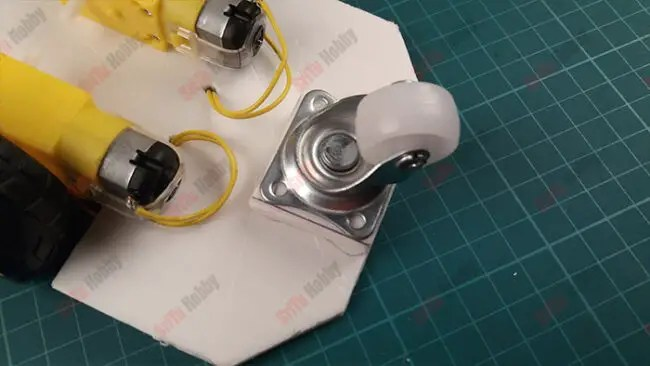### Step 5

Next, glue the motor driver board to the top of the chassis. After, connect these motors to the motor driver board. To do this, use the circuit diagram below.

### Step 6

Afterward, glue the four IR sensors to the robot chassis as follows.### Step 7

Now, glue the IR sensor module to the robot chassis.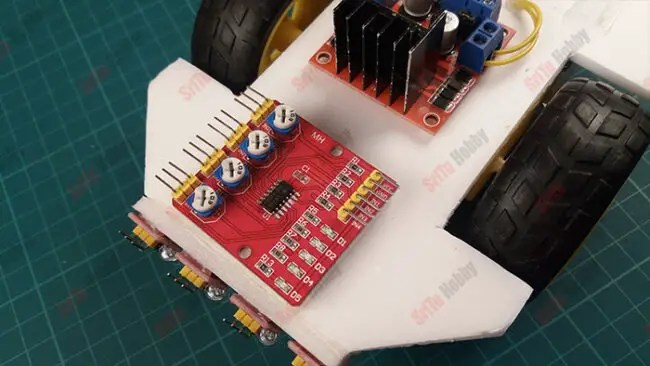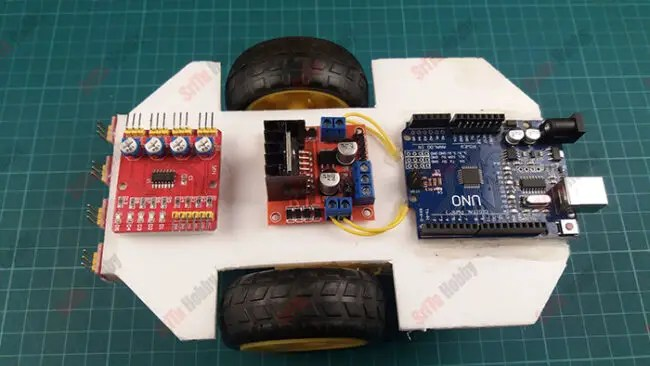### Step 8

Afterward, connect the IR module and motor driver board to the Arduino board. To do this, use the circuit diagram above.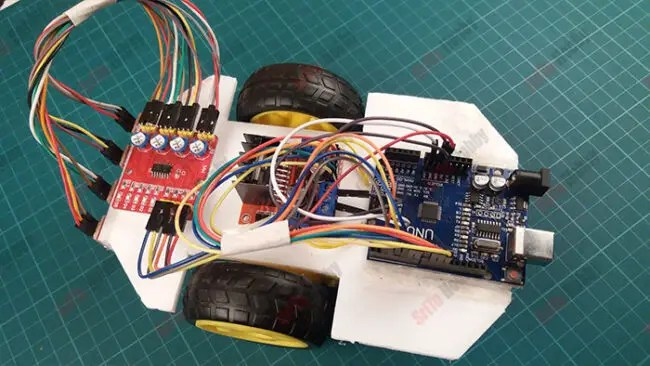### Step 9

Then, glue a foam board piece to the top of the Arduino board and glue the li-ion battery holder to it. After, put the batteries.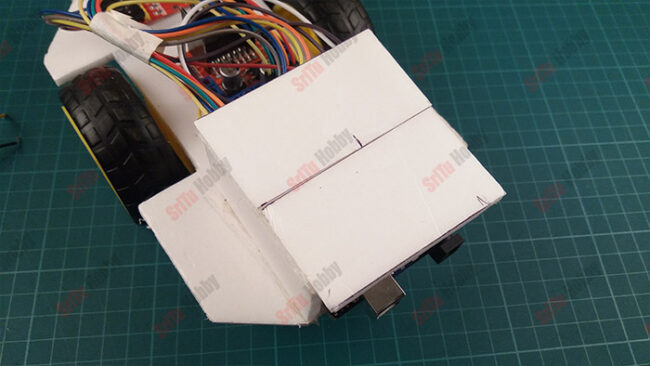### Step 10

Now, connect this robot to the computer. OK, let’s creates the program for this robot. It is as follows.

``````//Motor one
#define ENA 6
#define IN1 7
#define IN2 8

//Motor two
#define IN3 9
#define IN4 10
#define ENB 11

#define Speed 100

void setup() {
//Sensor pins
pinMode(2, INPUT);
pinMode(3, INPUT);
pinMode(4, INPUT);
pinMode(5, INPUT);
//Motor one
pinMode(ENA, OUTPUT);
pinMode(IN1, OUTPUT);
pinMode(IN2, OUTPUT);
//Motor two
pinMode(ENB, OUTPUT);
pinMode(IN3, OUTPUT);
pinMode(IN4, OUTPUT);

}
void loop() {

if (SOne == 0 && STwo == 1 && SThree == 1 && SFour == 0 ) {
forward();
} else if (SOne == 1 && STwo == 1 && SThree == 1 && SFour == 1) {
Stop();
} else if (SOne == 0 && STwo == 0 && SThree == 0 && SFour == 0) {
Stop();
}  else if (SOne == 1 && STwo == 1 && SThree == 0 && SFour == 0) {
turnleft();
} else if (SOne == 0 && STwo == 0 && SThree == 1 && SFour == 1) {
turnright();
}
}

void forward() {
analogWrite(ENA, Speed);
analogWrite(ENB, Speed);
digitalWrite(IN1, LOW);
digitalWrite(IN2, HIGH);
digitalWrite(IN3, HIGH);
digitalWrite(IN4, LOW);
}
void turnright() {
analogWrite(ENA, Speed);
analogWrite(ENB, Speed);
digitalWrite(IN1, HIGH);
digitalWrite(IN2, LOW);
digitalWrite(IN3, HIGH);
digitalWrite(IN4, LOW);
}
void turnleft() {
analogWrite(ENA, Speed);
analogWrite(ENB, Speed);
digitalWrite(IN1, LOW);
digitalWrite(IN2, HIGH);
digitalWrite(IN3, LOW);
digitalWrite(IN4, HIGH);
}
void Stop() {
analogWrite(ENA, 0);
analogWrite(ENB, 0);
digitalWrite(IN1, LOW);
digitalWrite(IN2, LOW);
digitalWrite(IN3, LOW);
digitalWrite(IN4, LOW);
}``````

#### Code explanation

Firstly, motor pins and robot speed defined.
//Motor one
#define ENA 6
#define IN1 7
#define IN2 8

//Motor two
#define IN3 9
#define IN4 10
#define ENB 11

#define Speed 100

In the setup function, sensor pins and motor pins are set as INPUT and OUTPUT pins.
void setup() {
//Sensor pins
pinMode(2, INPUT);
pinMode(3, INPUT);
pinMode(4, INPUT);
pinMode(5, INPUT);
//Motor one
pinMode(ENA, OUTPUT);
pinMode(IN1, OUTPUT);
pinMode(IN2, OUTPUT);
//Motor two
pinMode(ENB, OUTPUT);
pinMode(IN3, OUTPUT);
pinMode(IN4, OUTPUT);
}

In the loop function, the sensor values are read and put into four Boolean variables. Afterward, these values are checked using the IF condition. If the values of the four sensors are 1 or 0, the robot stops. Also, if the center sensor values are 1 and the side sensor values are 0, the robot moves forward. If the sensor one and two values are 1, the robot turns left. Also, if the sensor four and five values are 1, the robot moves right.
void setup() {
//Sensor pins
pinMode(2, INPUT);
pinMode(3, INPUT);
pinMode(4, INPUT);
pinMode(5, INPUT);
//Motor one
pinMode(ENA, OUTPUT);
pinMode(IN1, OUTPUT);
pinMode(IN2, OUTPUT);
//Motor two
pinMode(ENB, OUTPUT);
pinMode(IN3, OUTPUT);
pinMode(IN4, OUTPUT);

}

void loop() {

if (SOne == 0 && STwo == 1 && SThree == 1 && SFour == 0 ) {
forward();
} else if (SOne == 1 && STwo == 1 && SThree == 1 && SFour == 1) {
Stop();
} else if (SOne == 0 && STwo == 0 && SThree == 0 && SFour == 0) {
Stop();
} else if (SOne == 1 && STwo == 1 && SThree == 0 && SFour == 0) {
turnleft();
} else if (SOne == 0 && STwo == 0 && SThree == 1 && SFour == 1) {
turnright();
}
}

Then, the robot moves forward, turning left, turning right, and stop functions are created.
void forward() {
analogWrite(ENA, Speed);
analogWrite(ENB, Speed);
digitalWrite(IN1, LOW);
digitalWrite(IN2, HIGH);
digitalWrite(IN3, HIGH);
digitalWrite(IN4, LOW);
}
void turnright() {
analogWrite(ENA, Speed);
analogWrite(ENB, Speed);
digitalWrite(IN1, HIGH);
digitalWrite(IN2, LOW);
digitalWrite(IN3, HIGH);
digitalWrite(IN4, LOW);
}
void turnleft() {
analogWrite(ENA, Speed);
analogWrite(ENB, Speed);
digitalWrite(IN1, LOW);
digitalWrite(IN2, HIGH);
digitalWrite(IN3, LOW);
digitalWrite(IN4, HIGH);
}
void Stop() {
analogWrite(ENA, 0);
analogWrite(ENB, 0);
digitalWrite(IN1, LOW);
digitalWrite(IN2, LOW);
digitalWrite(IN3, LOW);
digitalWrite(IN4, LOW);
}

### Step 11

Lastly, select board and port. After, upload this code to the robot.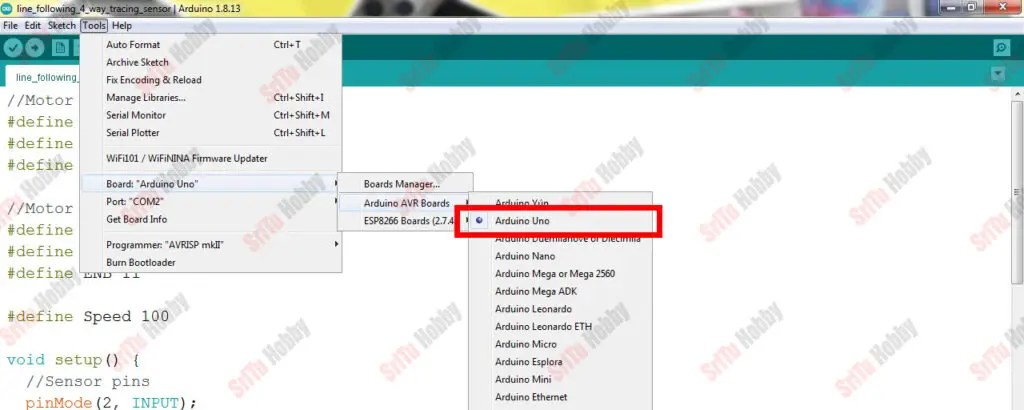OK, enjoy this project. For that, use a black track on a white surface. The full video guide is given below. So, we will meet in the next tutorial.

Shopping Cart